# Engineering Mechanics - Kinematics of Particle (KOP)

### Exercise :: Kinematics of Particle (KOP) - General Questions

• Kinematics of Particle (KOP) - General Questions
11.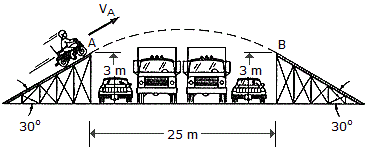The motorcyclist attempts to jump over a series of cars and trucks and lands smoothly on the other ramp, i.e., such that his velocity is tangent to the ramp at B. Determine the launch speed vA necessary to make the jump.

 A. vA = 11.90 m/s B. vA = 11.07 m/s C. vA = 16.83 m/s D. vA = 15.66 m/s

Explanation:

No answer description available for this question. Let us discuss.

12.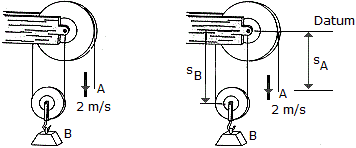If the end of the cable at A is pulled down with a speed of 2 m/s, determine the speed at which block B arises.

 A. vB = 4.00 m/sB. vB = 1.000 m/sC. vB = 1.000 m/sD. vB = 4.00 m/sExplanation:

No answer description available for this question. Let us discuss.

13.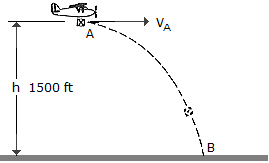A package is dropped from the plane which is flying with a constant horizontal velocity of vA = 150 ft/s at a height h = 1500 ft. Determine the radius of curvature of the path of the package just before it is released from plane at A.

 A.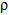A = 9860 ft B.A = 3000 ft C.A = 1500 ft D.A = 8510 ft

Explanation:

No answer description available for this question. Let us discuss.

14.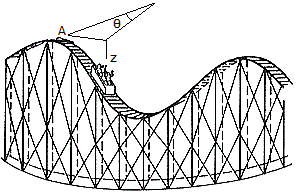For a short time the position of a roller-coaster car along its path is defined by the equations r = 25 m,= (0.3t) rad, and z = (-8 cos) m, where t is measured in seconds, Determine the magnitudes of the car's velocity and acceleration when t = 4s.

 A. v = 7.83 m/s, a = 2.27 m/s2 B. v = 9.74 m/s, a = 2.51 m/s2 C. v = 7.50 m/s, a = 2.25 m/s2 D. v = 5.26 m/s, a = 1.989 m/s2

Explanation:

No answer description available for this question. Let us discuss.

15.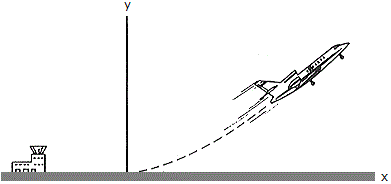The flight path of a jet aircraft as it takes off is defined by the parmetric equations x = 1.25 t2 and y = 0.03 t3, where t is the time after take-off, measured in seconds, and x and y are given in meters. At t = 40 s (just before it starts to level off), determine at this instant (a) the horizontal distance it is from the airport, (b) its altitude, (c) its speed and (d) the magnitude of its acceleration.

 A. x = 2.00 km, y = 1.92 km, v = 100.0 m/s, a = 2.50 m/s2 B. x = 2.00 km, y = 1.92 km, v = 144.0 m/s, a = 7.20 m/s2 C. x = 2.00 km, y = 1.92 km, v = 175.0 m/s, a = 7.62 m/s2 D. x = 2.00 km, y = 1.92 km, v = 32.9 m/s, a = 7.90 m/s2